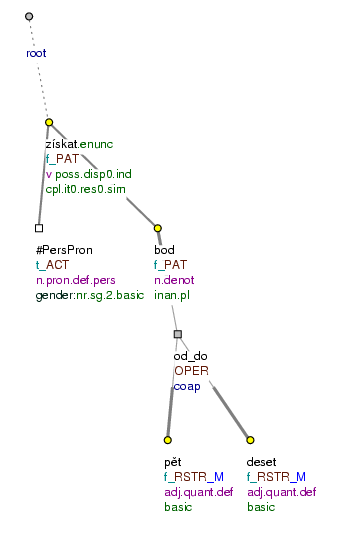### 6.3. Connecting elements when expressing mathematical operations and intervals

Also some connections of elements expressing mathematical operations and intervals are represented in the form of a paratactic structure - even if such a connection has a hypotactic form (see Section 16.2, "Operators").

Compare:

• Můžeš získat od pěti po deset bodů. (=lit. (You) can get from five to ten points.)

The interval pět - deset (=lit. five - ten) is represented as a paratactic structure in the tectogrammatical tree, even though hypotactic means are used. The root node of the paratactic structure is represented by the node for the operator od - po (=lit. from - to) (`t_lemma`=od_do; `functor`=`OPER`). The nodes representing the members of the interval (`t_lemma`=pět, `t_lemma`=deset) are the terminal elements of the paratactic structure. The value of the `is_member` attribute is `1`. Cf. Fig. 6.104.

These constructions are described in detail in Section 11, "Mathematical operations and intervals".

Figure 6.104. Expressing an intervalMůžeš získat od pěti po deset bodů. (=lit. (You) can get from five to ten points.)